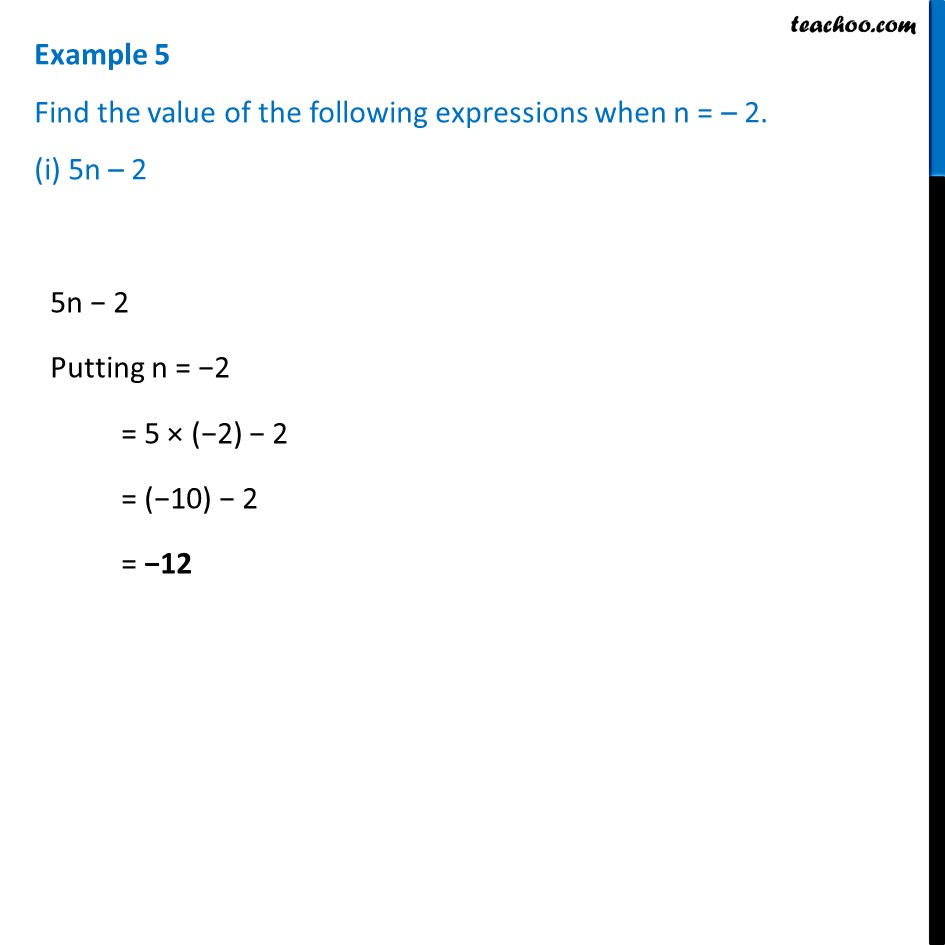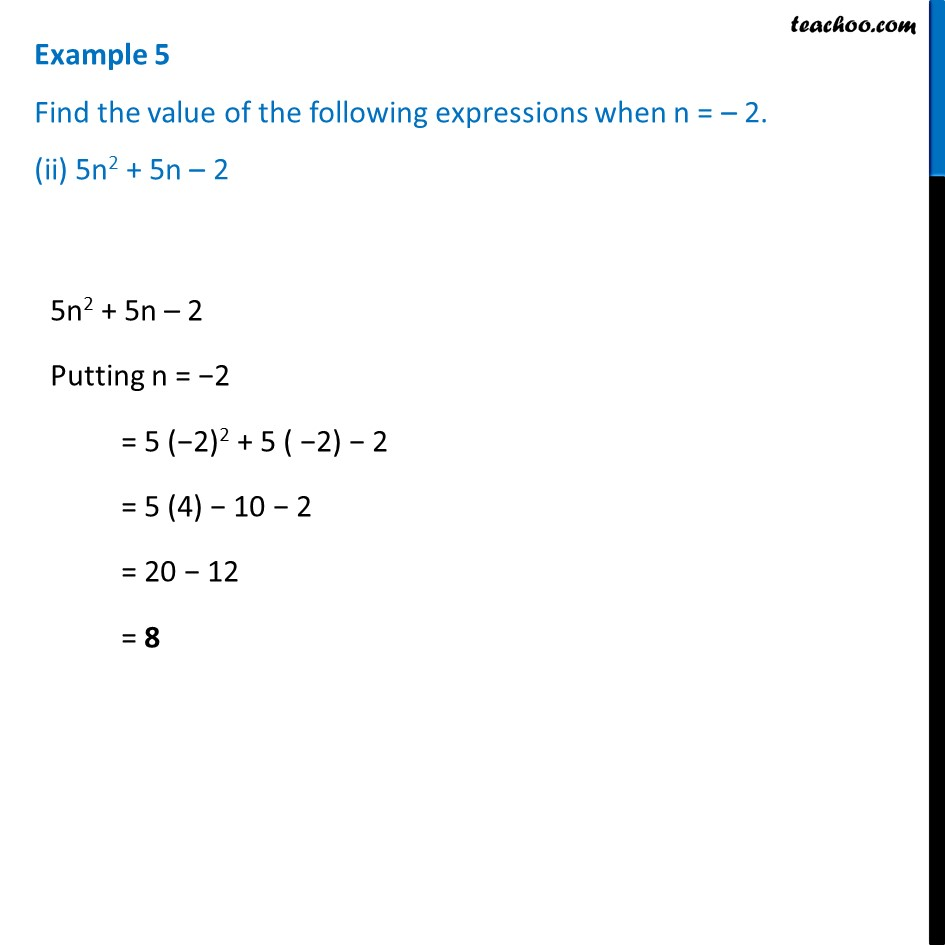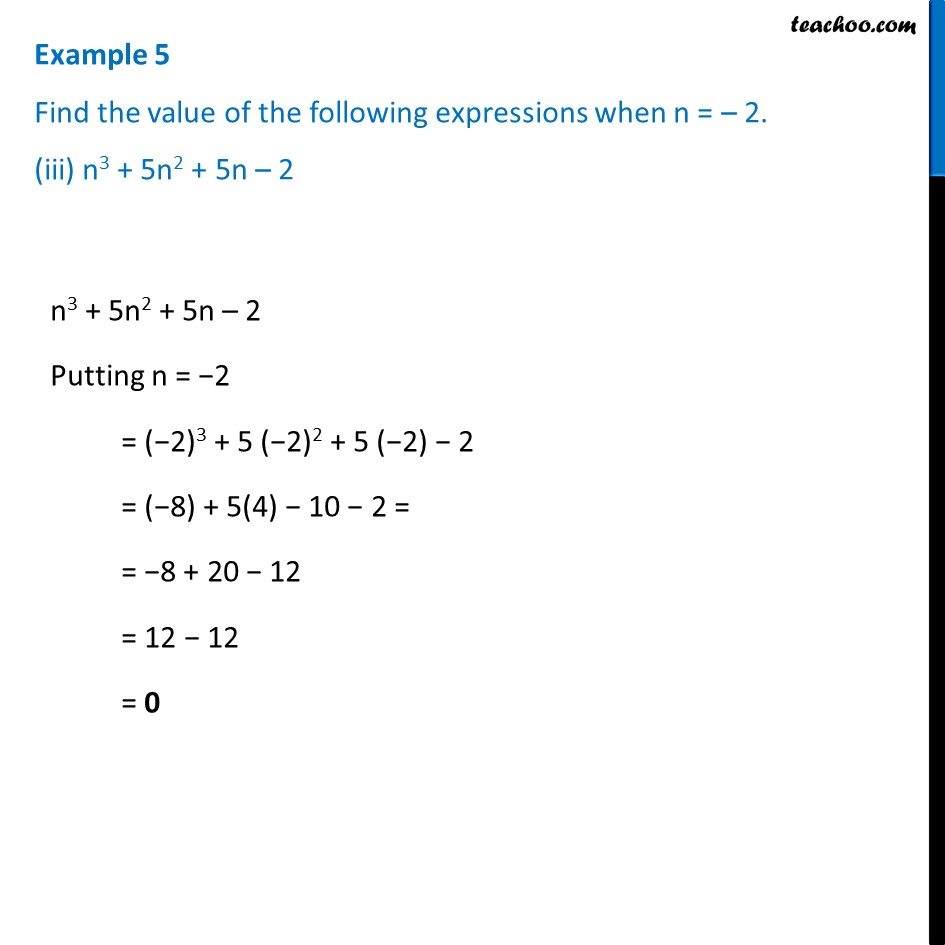Examples

Chapter 10 Class 7 Algebraic Expressions
Serial order wiseLearn in your speed, with individual attention - Teachoo Maths 1-on-1 Class

### Transcript

Example 5 Find the value of the following expressions when n = – 2. (i) 5n – 2 5n − 2 Putting n = −2 = 5 × (−2) − 2 = (−10) − 2 = −12 Example 5 Find the value of the following expressions when n = – 2. (ii) 5n2 + 5n – 2 5n2 + 5n – 2 Putting n = −2 = 5 (−2)2 + 5 ( −2) − 2 = 5 (4) − 10 − 2 = 20 − 12 = 8 Example 5 Find the value of the following expressions when n = – 2. (iii) n3 + 5n2 + 5n – 2n3 + 5n2 + 5n – 2 Putting n = −2 = (−2)3 + 5 (−2)2 + 5 (−2) − 2 = (−8) + 5(4) − 10 − 2 = = −8 + 20 − 12 = 12 − 12 = 0